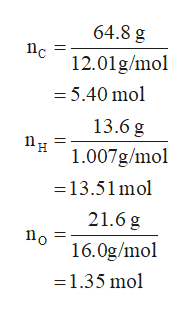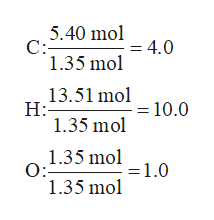Determine the empirical formula for a compound that is 64.8% C, 13.6% H, and 21.6% O by mass.

Question

Determine the empirical formula for a compound that is 64.8% C, 13.6% H, and 21.6% O by mass.

Step 1

Empirical formula of a compound is the simplest ratio of the elements present in a particular compound. The steps involved in the determination of the empirical formula of the compound are:

1. Calculate the number of moles of each element present in the compound.
2. Divide the number of moles of each element by the smallest value of number of moles.
3. Convert the obtained values into the nearest whole number ratio if the they are in fraction.

The number of moles of a species is calculated by expression (1) in which n is the number of moles, m is the mass and M is the molar mass of the species.

Step 2

As the given compound is 64.8 % C, 13.6 % H and 21.6 % O by mass, therefore, a 100 g sample of the given compound will have 64.8 g C, 13.6 g H and 21.6 g O.

The number of moles of C, H and O are calculated using equation (1),help_outlineImage Transcriptionclose64.8 g 12.01g/mol = 5.40 mol 13.6 g 1.007g/mol =13.51mol 21.6 g o 16.0g/mol 1.35 mol fullscreen
Step 3

Divide the number of moles of C, H and O by the number of moles of O because the value of the number of moles of oxyge...help_outlineImage Transcriptionclose5.40 mol4.0 C: 1.35 mol 13.51 mol 10.0 H: 1.35 mol 1.35 mol1,0 O: 1.35 mol fullscreen

Want to see the full answer?

See Solution

Want to see this answer and more?

Our solutions are written by experts, many with advanced degrees, and available 24/7

See Solution
Tagged in

Chemistry